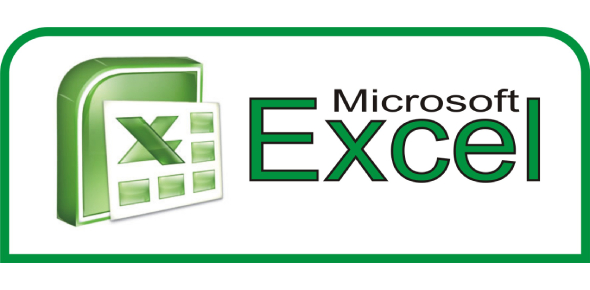# The Ultimate Microsoft Excel Quiz!

114 Questions | Attempts: 17586
ShareSettingsWelcome to the ultimate Microsoft Excel Quiz! Whether it’s for school, college, work or personal use, Excel is incredibly handy spreadsheet software that allows you to track and analyze data of all kinds. What do you know about the tool? Let’s take a look right now!

• 1.
The number of rows in an Excel 2007 worksheet is:
• A.

65536

• B.

256

• C.

64536

• D.

1048576

• 2.
You can have results of any database table into Excel Worksheet, and by one click you can refresh the results?
• A.

True

• B.

False

• 3.
The Insert Function dialog box tells you how to use functions.
• A.

True

• B.

False

• 4.
When a worksheet is printed, the grid lines that surround the cells are printable by default.
• A.

True

• B.

False

• 5.
When you clear a filter, all filters in the worksheet are cleared, if worksheet contains more than one filters
• A.

True

• B.

False

• 6.
The function arguments dialog box tells you how to use functions.
• A.

True

• B.

False

• 7.
The simplest and most convenient way to add a record to a table is to enter the data in the first blank row.
• A.

True

• B.

False

• 8.
Although 3D Charts are visually attractive, they can obscure the relationship between the values in the chart by making it difficult to see when slice is larger.
• A.

True

• B.

False

• 9.
Excel has a builtin format to display 4 digit year values, so you do not need to create one.
• A.

True

• B.

False

• 10.
Formatting data only change the appearance of data, it does not affect the data itself.
• A.

True

• B.

False

• 11.
If a formula contains several functions, Excel starts with the outermost function and then moves inward.
• A.

True

• B.

False

• 12.
An easy way to sort data when there is only one sort field is to use the Sort A to Z or Sort Z to A buttons.
• A.

True

• B.

False

• 13.
You can create maximum one Excel table in a worksheet
• A.

True

• B.

False

• 14.
As you begin to type a function name within a formula into a cell, a list of functions that begin with the letters you typed appears.
• A.

True

• B.

False

• 15.
You can rotate a 3D chart in only two directions: horizontally along the x-axis and vertically along the y-axis.
• A.

True

• B.

False

• 16.
Pressing ENTER moves the selection one cell to the right by default.
• A.

True

• B.

False

• 17.
Ctrl+B key makes the shortcut for making font in selected cell bold.
• A.

True

• B.

False

• 18.
What key should be used to edit the content of a cell?
• A.

F1

• B.

F2

• C.

F3

• 19.
What function offers you the possibility to view different results depending on the entered condition?
• A.

=If()

• B.

=Sum()

• C.

=SumIf()

• 20.
A user wishes to remove a worksheet from a workbook. Which is the correct sequence of events that will do this?
• A.

Go to file - save as - save as type - excel 4.0 worksheet

• B.

Right click on the worksheet tab and select delete

• C.

Right click on the worksheet and select insert - entire column

• 21.
Which formula can add the all numeric values in a range of cells, ignoring those which are not numeric, and place the result in a different cell?
• A.

Count

• B.

Average

• C.

Sum

• 22.
What is the keyboard shortcut for creating a chart from the selected cells?
• A.

F3

• B.

F7

• C.

F9

• D.

F11

• 23.
Using the autosum button will place in the selected cell _____.
• A.

The sum of values in the cell's column

• B.

Nothing until you selects a range of cells

• C.

The sum of the cell's row unless you change the range

• D.

A formula which will add values in the range Excel guesses you want to add

• 24.
The view that puts a blue line around each page that would be printed is the _____.
• A.

Print Preview

• B.

Normal

• C.

Page Break Preview

• D.

Split View

• 25.
The cell reference for a range of cells that starts in cell B1 and goes over to column G and down to row 10 is _____.
• A.

B1-G10

• B.

B1.G10

• C.

B1;G10

• D.

B1:G10

## Related TopicsBack to top
×

Wait!
Here's an interesting quiz for you.